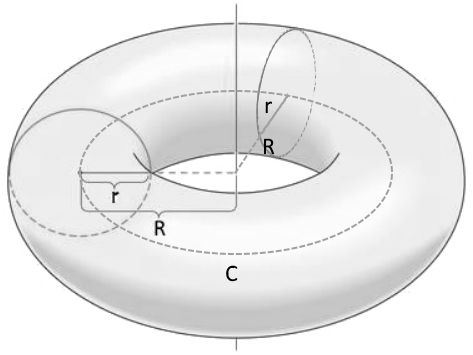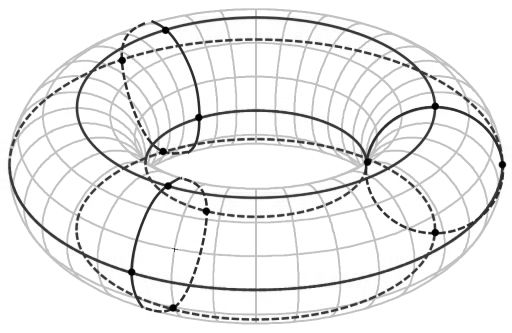시간 제한 메모리 제한 제출 정답 맞은 사람 정답 비율
1 초 512 MB 0 0 0 0.000%

## 문제

Torus is a surface that composed of points at a distance of $r$ from a circle $C$ with radius $R$ in 3D space. $C$ is then called a central circle of a torus; line perpendicular to the plane of $C$ that also contais the center of $C$ is called torus' axis; $R$ and $r$ are major and minor torus radiuses, accordingly. A circle on a torus is called bigger if it's center is on torus' axis and lesser if it's radius is $r$ and it's plane contains torus' axis.

Young traveler Senya lives on a torus-shaped planet with major radius $R$ and minor radius $r$. There is a regular road map on this planet: $n$ lesser roads at equal distance from each other (located on lesser circles), and $4$ bigger roads (on bigger circles): outer --- the most distant from the axis, inner --- the least distant one, and also nothern and southern equal to the central circle on the opposite sides of the planet.

Each lesser road is a property of one of $n$ countries. Each country has only $4$ cities on the intersections of it's lesser road with all $4$ bigger roads.Left picture illustrates the definition and shows minor and major radiuses. The right one shows $4$ greater roads and $n = 3$ lesser roads with cities on their intersections.

Senya wants to become The Great Traveler, which means that he wants to visit every country on the planet. He considers a country visited if he has traveled along the road between two different cities of this country. Of course, movement on his planet is allowed only by the roads.

Please, help Senya to find the distance he need to cover to become The Great Traveler, if he starts his journey in a city on the inner road.

## 입력

The first line of input contains three integers: $r$, $R$ --- minor and major torus radiuses and $n$ --- number of countries ($1 \le r < R \le 10^9$; $1 \le n \le 10^9$).

## 출력

Output should contain one real number --- minimal distance Senya has to cover. Your answer should have an absolute or relative error not greater than $10^{-9}$.

## 예제 입력 1

1 2 1


## 예제 출력 1

1.570796327


## 예제 입력 2

1 3 4


## 예제 출력 2

18.849555922


## 힌트

In the second example let $i_k, s_k, n_k, o_k$ represent the cities of $k$-th country on the inner, southern, nothern and outer roads, accordingly. One of the minimal paths looks like: $i_1 \rightarrow s_1 \rightarrow s_2 \rightarrow i_2 \rightarrow i_3 \rightarrow n_3 \rightarrow i_3 \rightarrow i_4 \rightarrow s_4$. Covered distance equals to $\frac{5}{4}$ of country length, two segments of inner road and one of southern. So the answer is $\frac{5}{4} \cdot 2 \pi \cdot 1 + 2 \cdot \frac{2 \pi \cdot 2}{4} + \frac{2 \pi \cdot 3}{4} = 6 \pi \approx 18.849555922$.